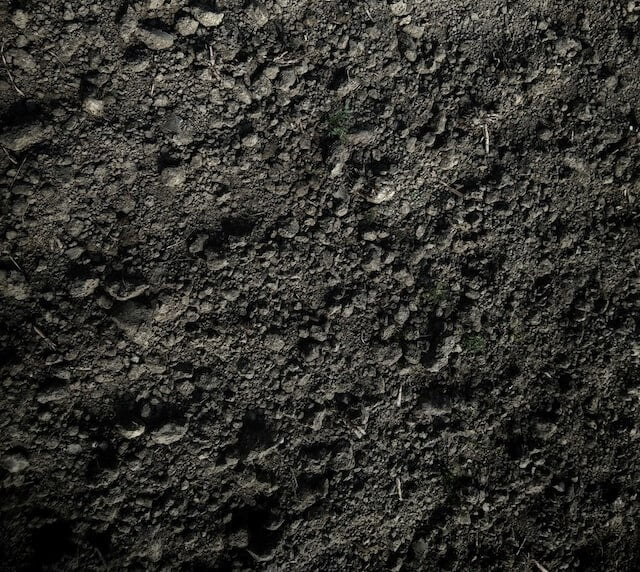Category: consolidation

# The Utility of Consolidation Data

The Utility of Consolidation Data The consolidation data is used for the following purpose: 1. Safe engineering design of structures.2. Forecasting of the probable settlement of a structure.3. Solving of various problems connected with the settlement of structures due to consolidation.

# 9 Assumption in Terzaghi Theory of Consolidation

Terzaghi Theory of Consolidation The theoretical concept of consolidation process was developed by Terzaghi. In order to compute the amount of consolidation( or settlement) after any given time t, Terzaghi derived the following basic equation is a free surface. δu/δt = cv (δu/δz) Where, cv = Coefficient of consolidation.   The above differential equation of consolidation … Continue reading 9 Assumption in Terzaghi Theory of Consolidation# Consolidation Settlement Analysis – Soil

Consolidation Settlement Analysis In one dimensional consolidation, the change in the height(ΔH) (i.e settlement) per unit of original height(H) equals to the change in volume(ΔV) per unit of the original volume(V). ΔH/H = ΔV/V .............(1).   Consolidation Settlement (Settlement Analysis) Now, at an initial condition, void ratio(e0) = Vv/Vs Or Vv= e0.Vs Where Vv and Vs … Continue reading Consolidation Settlement Analysis – Soil

# What is the Coefficient of Consolidation, Compressibility and Volume change With Their Equation

Coefficient of Consolidation The term coefficient of consolidation() is used to indicate the combined effects of the permeability and compressibility of soil on the rate of volume change. It is the ratio of the coefficient of permeability(K) to the product of the coefficient of volume compressibility() and unit weight of water( ). Equation of The … Continue reading What is the Coefficient of Consolidation, Compressibility and Volume change With Their Equation# What is Consolidation and Consolidation Factors?

What is Consolidation? Consolidation can be defined as the compression resulting from a long term static load and the consequent escape of pore water from the saturated soil mass. According to terzaghi, every process involving a decrease in the water content of a saturated soil mass due to the replacement of water by air is … Continue reading What is Consolidation and Consolidation Factors?